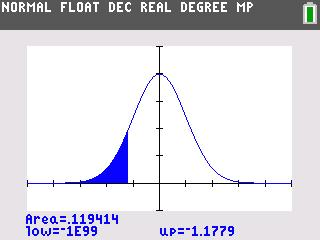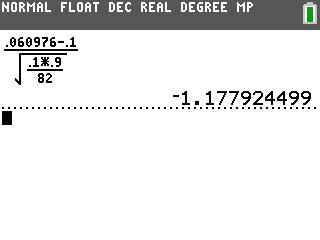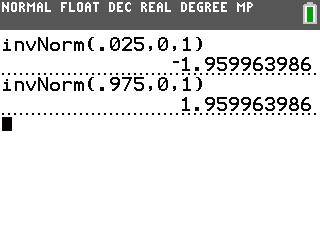# Activities

••• ##### Subject Area

• Math: Statistics: Inferential Statistics

• ##### Author9-12

40 Minutes

• ##### Device
• TI-83 Plus Family
• TI-84 Plus
• TI-84 Plus Silver Edition
•TI-84 Plus C Silver Edition
•TI-84 Plus CE
• ##### Report an Issue#### Activity Overview

Students find z-scores and critical values to test claims about proportions.

#### Key Steps

•In Problem 1, students begin hypothesis testing. They write null and alternative hypotheses for a two-tailed test. They test the claim that 10% of Americans are left-handed.

•Students then calculate the critical values. They will determine that because the normal distribution is symmetric, they can find the left critical value using the Inverse Normal (invNorm) command. and use its opposite for the right critical value.

Students discuss whether to reject or fail to reject the null hypothesis based on the test statistic and the critical values.

•Students then calculate the critical values. They will determine that because the normal distribution is symmetric, they can find the left critical value using the Inverse Normal (invNorm) command. and use its opposite for the right critical value.

Students discuss whether to reject or fail to reject the null hypothesis based on the test statistic and the critical values.